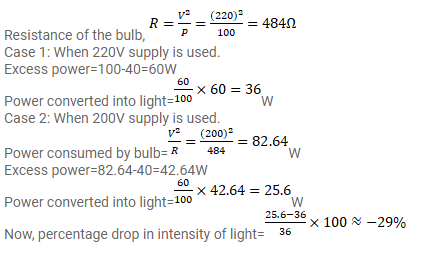# The coil of an electric bulb takes 40 watts to start glowing.

Question:

The coil of an electric bulb takes 40 watts to start glowing. If more than $40 \mathrm{~W}$ is supplied, $60 \%$ of the extra power is converted into light and the remaining into heat. The bulb consumes $100 \mathrm{~W}$ at $220 \mathrm{~V}$. Find the percentage drop in the light intensity at a point if the supply voltage changes from $220 \mathrm{~V}$ to $200 \mathrm{~V}$.

Solution: